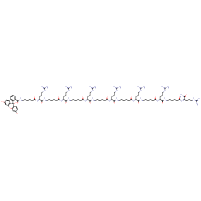Structure InformationCompound Identification

SMILES

NC(=N)NCCC[C@@H](NC(=O)CCCCCNC(=O)[C@@H](CCCNC(N)=N)NC(=O)CCCCCNC(=O)[C@@H](CCCNC(N)=N)NC(=O)CCCCCNC(=O)[C@@H](CCCNC(N)=N)NC(=O)CCCCCNC(=O)[C@@H](CCCNC(N)=N)NC(=O)CCCCCNC(=O)[C@@H](CCCNC(N)=N)NC(=O)CCCCCNC(=O)[C@@H](CCCNC(N)=N)NC(=O)CCCCCNC(=O)C1=CC=CC=C1C1(O)C2=C(OC3=C1C=CC(O)=C3)C=C(O)C=C2)C(N)=O

InChIKey

InChIKey=MORSFOGAGPPYCA-PFXOJAAHSA-N

Formula

C104H176N36O19

Mass

2234.785

### Export to:

Taxonomic Classification

Taxonomy Tree

Kingdom

Organic compounds

Superclass

Organic acids and derivatives

Class

Carboxylic acids and derivatives

Subclass

Amino acids, peptides, and analogues

Intermediate Tree Nodes

Amino acids and derivatives - Alpha amino acids and derivatives

Direct Parent

Arginine and derivatives

Alternative Parents

Molecular Framework

Aromatic heteropolycyclic compounds

Substituents

Arginine or derivatives - Dibenzopyran - Xanthene - N-acyl-alpha amino acid or derivatives - Alpha-amino acid amide - Diaryl ether - Benzopyran - 1-benzopyran - Benzamide - Benzoic acid or derivatives - Benzoyl - Phenol - 1-hydroxy-2-unsubstituted benzenoid - Fatty acyl - Monocyclic benzene moiety - Fatty amide - N-acyl-amine - Benzenoid - Tertiary alcohol - Carboxamide group - Secondary carboxylic acid amide - Primary carboxylic acid amide - Guanidine - Ether - Carboximidamide - Organoheterocyclic compound - Oxacycle - Polyol - Organic oxygen compound - Organic nitrogen compound - Alcohol - Carbonyl group - Hydrocarbon derivative - Organic oxide - Imine - Organooxygen compound - Organonitrogen compound - Aromatic heteropolycyclic compound

Description

This compound belongs to the class of organic compounds known as arginine and derivatives. These are compounds containing arginine or a derivative thereof resulting from reaction of arginine at the amino group or the carboxy group, or from the replacement of any hydrogen of glycine by a heteroatom.

External Descriptors

Not available